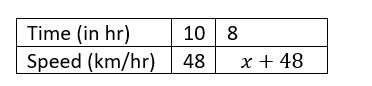Home/Class 8/Maths/

A car can finish a certain journey in 10 hours at the speed of 48 km/hr. By how much should its speed be increased so that it may take only 8 hours to cover the same distance ? (in km/hr)Speed
00:00
03:40## QuestionMathsClass 8

A car can finish a certain journey in 10 hours at the speed of 48 km/hr. By how much should its speed be increased so that it may take only 8 hours to cover the same distance ? (in km/hr)

$$12\,km/hr$$
4.64.6## Solution

Let the speed increased by $$x$$ km/hr.
So increased speed $$=(x+48)$$km/hrSince speed and time taken are in inverse variation, we get:
$$\Rightarrow$$ $$10\times 48=8(x+48)$$
$$\Rightarrow$$ $$480=8x+384$$
$$\Rightarrow$$ $$8x=480-384$$
$$\Rightarrow$$ $$8x=96$$
$$\Rightarrow$$ $$x=12$$
$$\therefore$$ The speed should be increased by $$12\,km/hr$$